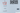# Leetcode - Rearrange Spaces Between Words

October 06, 2020## Problem Statement

You are given a string text of words that are placed among some number of spaces. Each word consists of one or more lowercase English letters and are separated by at least one space. It’s guaranteed that text contains at least one word.

Rearrange the spaces so that there is an equal number of spaces between every pair of adjacent words and that number is maximized. If you cannot redistribute all the spaces equally, place the extra spaces at the end, meaning the returned string should be the same length as text.

Return the string after rearranging the spaces.

Example

``````Input: text = "  this   is  a sentence "
Output: "this   is   a   sentence"

Input: text = " practice   makes   perfect"
Output: "practice   makes   perfect "

Input: text = "hello   world"
Output: "hello   world"``````

## Solution

You need to have three things to solve this problem:

1. Number of words
2. The actual words
3. Number of spaces

If you have 4 words, and 9 spaces. You will put `9 / (4-1) = 3` spaces in between.

Lets look at the algorithm:

• First get the number of words, number of spaces, and the words
• Calculate how many spaces you will put in between words
• Calculate how many remainder spaces you will need to put after final string
• There are some special conditions when
• there is only spaces
• there is one word and none or few spaces

### Code

Lets look at the code now

``````private int process(String text, List<String> words) {
int spaces = 0;

for (int i=0; i<text.length(); ) {
if (text.charAt(i) == ' ') {
while (i < text.length() && text.charAt(i) == ' ') {
spaces ++;
i++;
}
}
else {
StringBuilder sb = new StringBuilder();
while (i < text.length() && text.charAt(i) != ' ') {
sb.append(text.charAt(i));
i++;
}
if (sb.length() > 0) {
}
}
}

return spaces;
}

public String reorderSpaces(String text) {
List<String> words = new ArrayList<String>();
int spaces = this.process(text, words);

int divider = (words.size() - 1) > 0 ? (words.size() - 1) : 1;

int targetSpaces = spaces / divider;
int remainingSpaces = spaces % divider;
if (words.size()<= 1) {
remainingSpaces += targetSpaces;
}

StringBuilder sb = new StringBuilder();
if (words.size() > 0) {
sb.append(words.get(0));
}
for (int i=1; i<words.size(); i++) {
for (int j=0; j<targetSpaces; j++) {
sb.append(" ");
}
sb.append(words.get(i));
}

if (remainingSpaces > 0) {
for (int j=0; j<remainingSpaces; j++) {
sb.append(" ");
}
}

return sb.toString();
}``````

### Complexity

Its `O(n)`